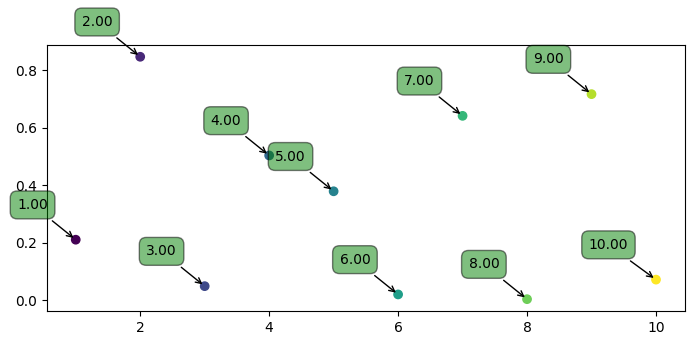# How to improve the label placement for Matplotlib scatter chart?

To imporove the label placement for matplotlib scatter chart, we can first plot the scatter points and annotate those points with labels.

## Steps

• Create points for and using numpy.

• Create labels using xpoints.

• Use scatter() method to scatter points.

• Iterate the labels, xpoints and ypoints and annotate the plot with label, x and y with different properties.

• To display the figure, use show() method.

## Example

import numpy as np
from matplotlib import pyplot as plt
plt.rcParams["figure.figsize"] = [7.00, 3.50]
plt.rcParams["figure.autolayout"] = True
xpoints = np.linspace(1, 10, 10)
ypoints = np.random.rand(10)
labels = ["%.2f" % i for i in xpoints]
plt.scatter(xpoints, ypoints, c=xpoints)
for label, x, y in zip(labels, xpoints, ypoints):
plt.annotate(
label,
xy=(x, y), xytext=(-20, 20),
textcoords='offset points', ha='right', va='bottom',
plt.show()